# Creating relative named ranges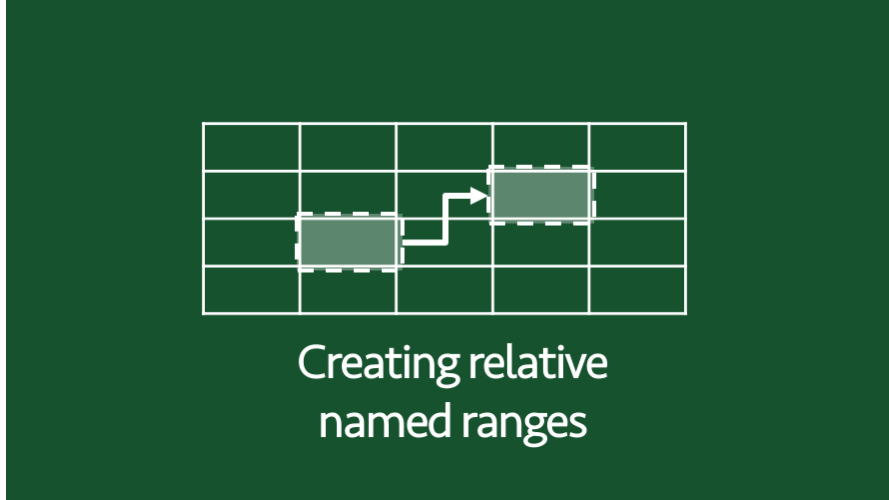I’ve posted many times about named ranges in various guises.  However, one thing I have not covered is relative named ranges.  Relative named ranges don’t sound too exciting, but once you’ve read this post I’m sure there will be plenty of examples where you could have used them and now wish you had.

## Creating named ranges

To start, let’s remind ourselves how to create a standard named range.  There are many ways to achieve this, we’ll go the long way, because creating a relative named range follows the same process.

From the Formulas ribbon click Name Manager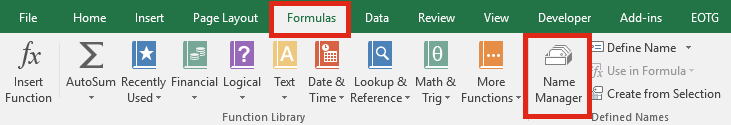The Name Manager window will open.  Click New.The New Name window appears.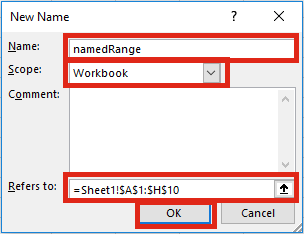The important options are:

• Name – the name you wish to give the named range
• Scope – this has two options, Workbook or Worksheet.
• A Workbook level named range can be used in a cell or calculation by using only its name.
• A Worksheet level named range must use the name of the sheet and the name of the name range if it is used outside the sheet in which the named range is held.
• Refers to – the range of cells (or can be a calculation) which you want to name.

Set these options then click OK.

Using the example in the screenshot above, any time =namedRange is used, it is equivalent to entering =Sheet1\$A\$1:\$H\$10.

## Creating relative named ranges

Excel will try to enter \$ signs (to freeze the cell references) automatically, but there is no requirement to use \$ symbols.  A named range can be created where the Refers to value is =Sheet1!A10:H10.  Without the \$ signs it has now become a relative named range.  The range is set relative to the cell selected at the time of creating the named range.

To prove how this works, I selected Cell E4, then I created a named range with Cells A1-B5 (without any \$ signs)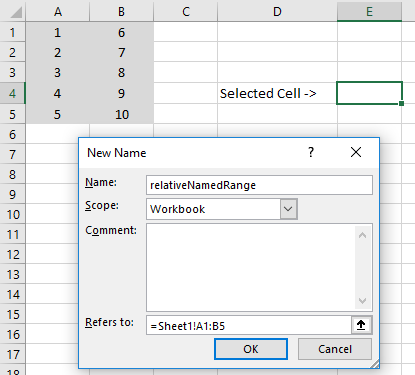By using this named range in a formula one cell below the cell selected at the time of creation, the named range will also move one cell down.The minimum value within the named range at creation would have been 1 (Cell A1), but the result of the calculation in the screenshot above is 2 (not 1).  The relative named range no longer contains the 1, as the cells have shifted down by one cell.

It is possible to mix and match the \$ symbols to freeze specific columns or rows.

## Uses for relative named ranges

That all sounds interesting, but is it actually useful?  Here are a few ideas to implement relative named ranges.

### Always referring to the cell above

If you have a formula which should always refer to the cells above, what happens when a new row is added?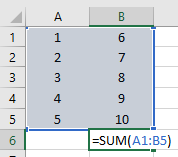The range of the formula does not change.  The formatting has copied down, but the formula has not.Creating a relative named range for the cell above would solve this problem.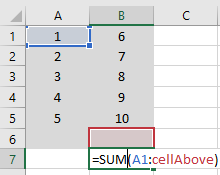It does not matter how many rows are added the formula will always refer to the cell directly above the formula.

### Simplifying calculations

One of the key advantages of named ranges is simplifying calculations.  This, rather basic example shows that a Total Calculation can be created and copied down.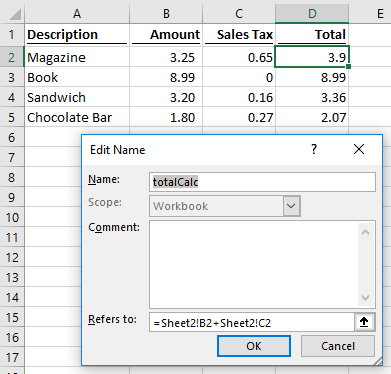The formulas in Cells D2 – D5 are all =totalCalc.  Excel has calculated a different result for each use of the named range.

### Running total

Creating a running total is also possible with a relative named range.  This example shows it is possible to mix relative and absolute cell references within a named range.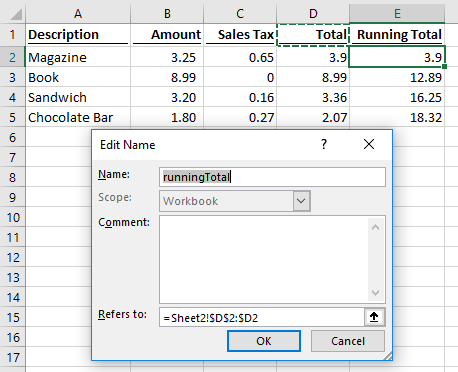The formulas in Cells E2 – E5 are =runningTotal.  Excel has calculated a different result for each use of the named range.

## A warning

It is not possible to create a ‘global’ relative named range.  In each of the examples above, the name of the sheet is used to define the named range, so there needs to be new relative named ranges for each worksheet.  I have seen suggestions of just using the “!” without the sheet name to make it ‘global’, however this can result in some strange behaviors and can even result in Excel crashing.  In such circumstances, it may be better to investigate using the INDIRECT or OFFSET functions.

## Want to Learn VBA & Macros?

If you want to automate Excel and save time, my recommended resouces for learning VBA Macros are:

### Books:

Excel 2016 Power Programming with VBA